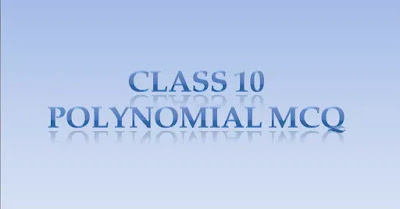# MCQ Questions class 10 math chapter polynomials 2021## MCQ Questions class 10 math chapter polynomials 2021

These questions are based on the latest MCQ pattern for the class 10 Term 1 board exam. This year-term 1 exam will be MCQ-based and will be given in OMR  sheets by students.

## Deleted portion for polynomials for board exams

Statement and simple problems on division algorithm for polynomials with real coefficients.

### Class 10 math polynomial questions

1. If  `alpha`,`B` are zeroes of `x^2`-4`x`+1 , then `frac1alpha+frac1B-alpha B` is :

(a) 3

(b) 5

(c) -5

(d) -3

2. The zeroes of the quadratic polynomial `x^2`-11`x`+28 are:

(a) Both positive

(b) Both negative

(c) One positive and one negative

(d) Both equal

3. If  `alpha`,`B` are zeroes of polynomial `fleft(xright)`= `x^2+px+q` then polynomial having `frac1alpha` and `frac1B` as its zeroes is :

(a) `x^2`+q`x`+p

(b) `x^2`-p`x`+q

(c) q`x^2`+p`x`+1

(d) p`x^2`+q`x`+1

4. The quadratic polynomial p(`x`) with -81 and 3 as the product and one of the zeroes respectively is:

(a) `x^2`+24`x`=81

(b) `x^2`-24`x`-81

(c) `x^2`-24`x`+81

(d) `x^2`+24`x`+81

5. If `alpha` and `B` are the zeroes of the polynomial 5`x^2`-7`x`+2 , then the sum of their reciprocals is:

(a) `frac72`

(b)`frac25`

(c) `frac75`

(d) `frac 1425`

6. If 1 is zero of the polynomial p(`x`) = `a“x^2`-3(`a`-1) `x`-1, then the value of  ‘a’ is :

(a) 2

(b) 1

(c) 3

(d) -3

7. The degree of the polynomial (`x+1` )(`x^2-x-x^4+1`) is:

(a) 1

(b) 2

(c) 4

(d) 5

8. The number of zeroes for the polynomial `y=p(x)`. If the graph meets the `x`-axis at the `3` point is

(a) 0

(b) 2

(c) 1

(d) 3

9. The number of polynomials having zeroes -2 and 4 is:

(a) 1

(b) 2

(c) 3

(d) more than 3

10. If `alpha` and `B` are zeroes of `x^2-6x+k`. What is the value of `k` if `3alpha+2B=20`

(a) 8

(b) -16

(c) 4

(d) -8

11. The zeroes of the polynomial `x^2-3x-4` are

(a) -3,-1

(b) -1,4

(c) -1,-4

(d) 2,-3

12. If `alpha` and `B` are the zeroes of the polynomial `x^2+5x+8` then the value of  `left(alpha+Bright)`  is

(a). -5

(b) 5

(c) -8

(d) 8

13. If `alpha` and `B` are the zeroes of `2x^2+5x-9` then the value of `alpha“B` is

(a) `frac{-5}4`

(b) `frac{-9}2`

(c) `frac{-7}4`

(d) `frac{-5}2`

14. If one zero of the quadratic polynomial `kx^2+3x+k` is 2 then the value of k is

(a) `frac{5}6`

(b) `frac{-9}2`

(c) `frac{-6}5`

(d) `frac{6}5`